Question

# If mean is 39.2 and standard deviation is 1.9, what is middle 70%

If mean is 39.2 and standard deviation is 1.9, what is middle 70%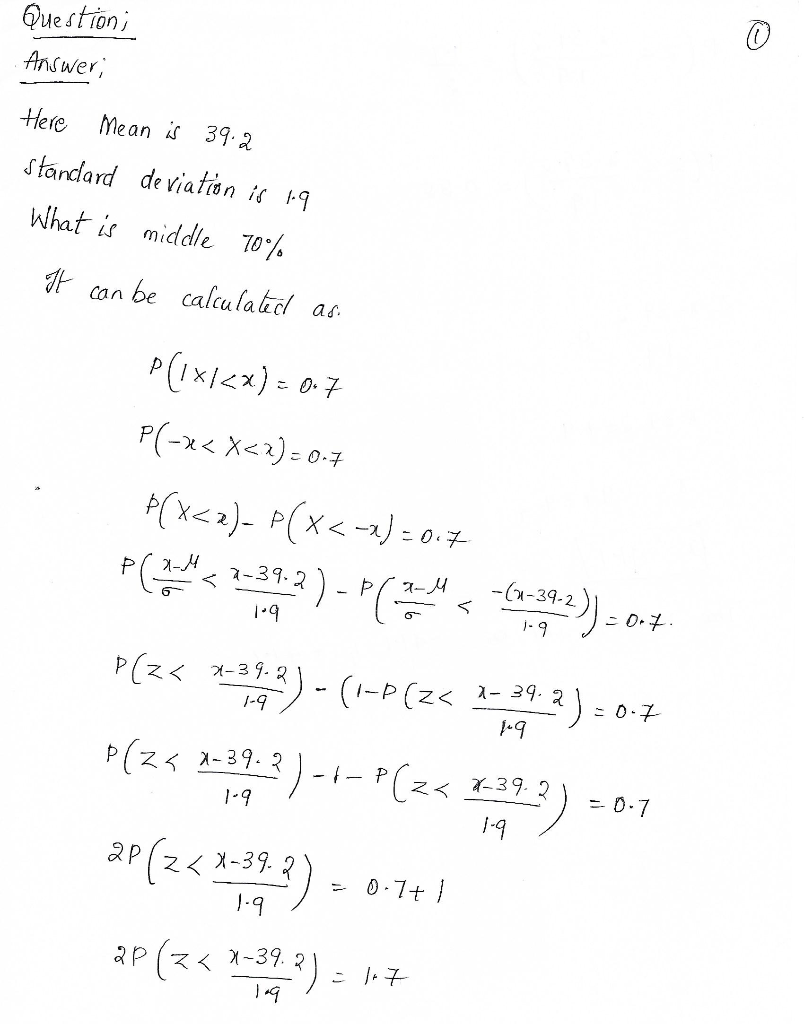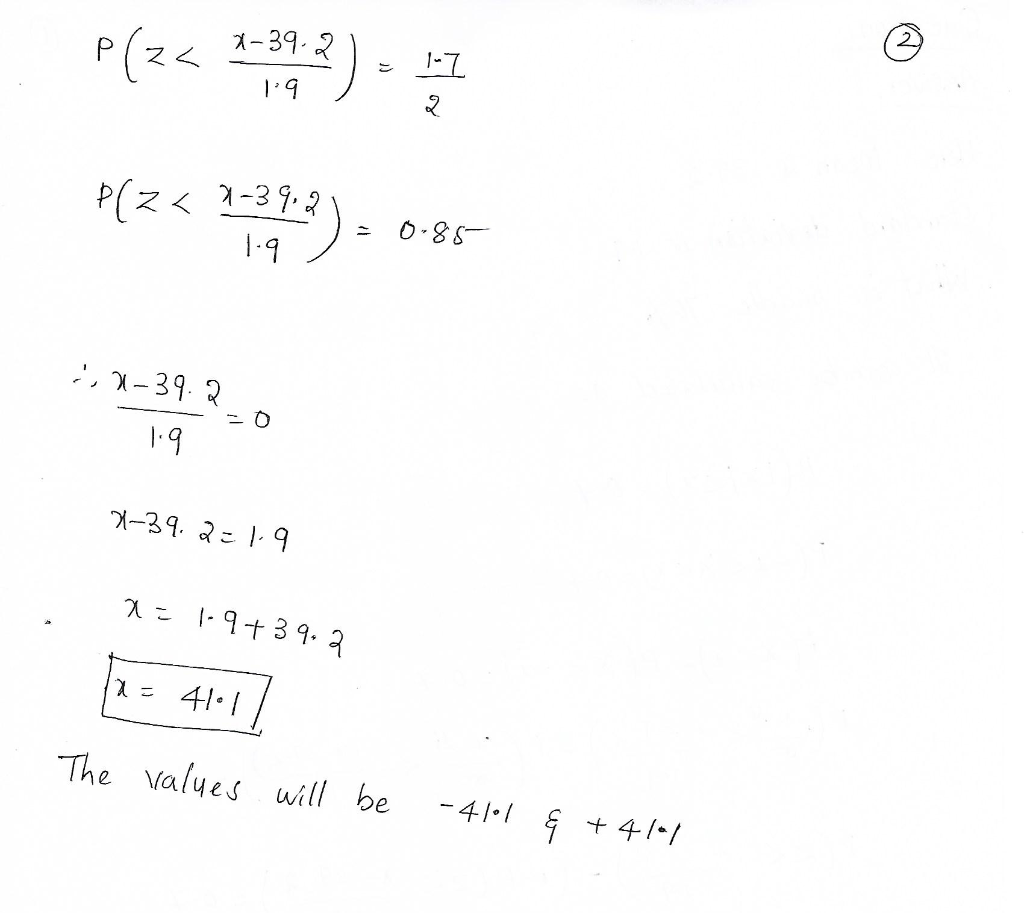#### Earn Coins

Coins can be redeemed for fabulous gifts.

Similar Homework Help Questions
• ### Scores on a test are normally distributed with a mean of 70 and standard deviation of...

Scores on a test are normally distributed with a mean of 70 and standard deviation of 10. Applying the Empirical Rule, we would expect the middle 95% of scores to fall between what two values? 40 and 100 50 and 90 55 and 85 60 and 80 65 and 75

• ### ATH241 23 In a sam standard deviation of 39.2. a.) What is the best point estimate...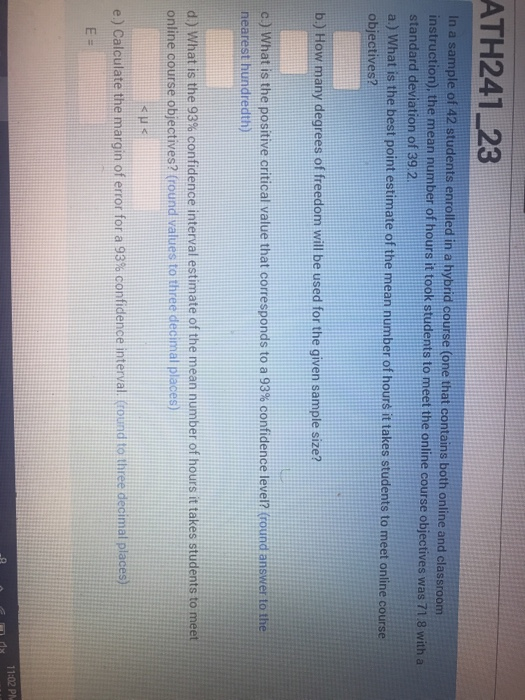ATH241 23 In a sam standard deviation of 39.2. a.) What is the best point estimate of the mean number of hours it takes students to meet online course ple of 42 students enrolled in a hybrid course (one that contains both online and classroom ). the mean number of hours it took students to meet the online course objectives was 71 8 with a objectives? b.) How many degrees of freedom will be used for the given sample size?...

• ### A distribution of values is normal with a mean of 230 and a standard deviation of...

A distribution of values is normal with a mean of 230 and a standard deviation of 25. From this distribution, you are drawing samples of size 27. Find the interval containing the middle-most 70% of sample means:

• ### Suppose x has a distribution with a mean of 70 and a standard deviation of 27....

Suppose x has a distribution with a mean of 70 and a standard deviation of 27. Random samples of size n = 36 are drawn. (a) Describe the x distribution and compute the mean and standard deviation of the distribution. x has distribution ___________ with mean μx = _______ and standard deviation σx = __________. (b) Find the z value corresponding to x = 79. z = (c) Find P(x < 79). (Round your answer to four decimal places.) P(x...

• ### A population has a mean of 300 and a standard deviation of 70. Suppose a sample...

A population has a mean of 300 and a standard deviation of 70. Suppose a sample of size 125 is selected and (x-bar) is used to estimate (mu) . Use z-table. A. What is the probability that the sample mean will be within +/- 8 of the population mean (to 4 decimals)? B. What is the probability that the sample mean will be within +/- 16 of the population mean (to 4 decimals)?

• ### A population has a mean of 200 and a standard deviation of 70, Suppose a sample...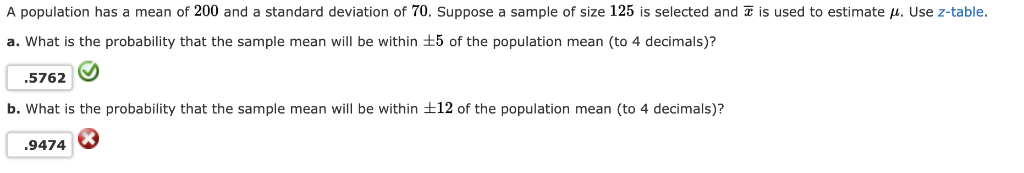A population has a mean of 200 and a standard deviation of 70, Suppose a sample of size 125 is selected and z is used to estimate μ. Use z-table a. What is the probability that the sample mean will be within :5 of the population mean (to 4 decimals)? 5762 b. What is the probability that the sample mean will be within t12 of the population mean (to 4 decimals)? 9474

• ### A population has a mean of 300 and a standard deviation of 70. Suppose a sample...

A population has a mean of 300 and a standard deviation of 70. Suppose a sample of size 100 is selected and is used to estimate What is the probability that the sample mean will be within +/- 3 of the population mean (to 4 decimals)? What is the probability that the sample mean will be within +/- 12 of the population mean (to 4 decimals)? (Round z value in intermediate calculations to 2 decimal places.)

• ### mean: 78, standard deviation:12 What proportion of adults have pulse rates less than 70?

mean: 78, standard deviation:12 What proportion of adults have pulse rates less than 70?

• ### The height of an adult male has a mean of 70 inches and a standard deviation...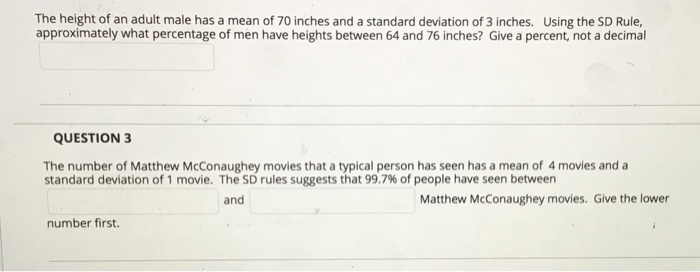The height of an adult male has a mean of 70 inches and a standard deviation of 3 inches. Using the SD Rule approximately what percentage of men have heights between 64 and 76 inches? Give a percent, not a decimal QUESTION 3 The number of Matthew McConaughey movies that a typical person has seen has a mean of 4 movies and a standard deviation of 1 movie. The SD rules suggests that 99.7% of people have seen between and...

• ### The mean age when smokers first start is 13 years old with a population standard deviation of 1.9 years.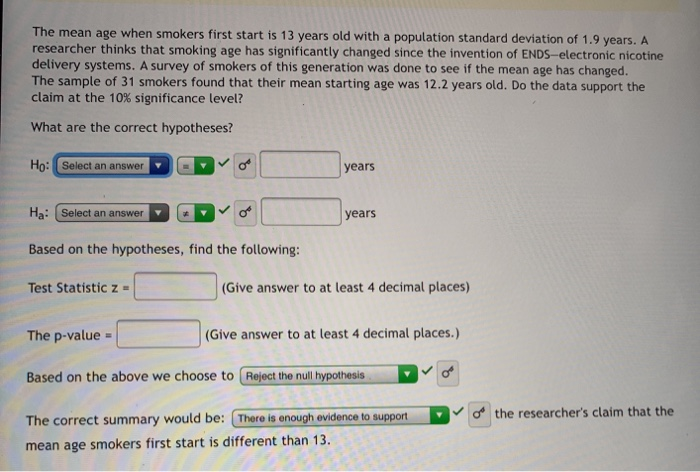The mean age when smokers first start is 13 years old with a population standard deviation of 1.9 years. A researcher thinks that smoking age has significantly changed since the invention of ENDS-electronic nicotine delivery systems. A survey of smokers of this generation was done to see if the mean age has changed. The sample of 31 smokers found that their mean starting age was 12.2 years old. Do the data support the claim at the 10% significance level? What are the...